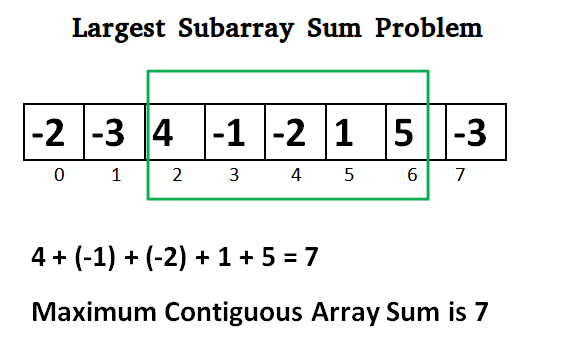C# Program for Largest Sum Contiguous Subarray

• Last Updated : 29 Nov, 2021

Write an efficient program to find the sum of contiguous subarray within a one-dimensional array of numbers that has the largest sum.Initialize:
max_so_far = INT_MIN
max_ending_here = 0

Loop for each element of the array
(a) max_ending_here = max_ending_here + a[i]
(b) if(max_so_far < max_ending_here)
max_so_far = max_ending_here
(c) if(max_ending_here < 0)
max_ending_here = 0
return max_so_far

Explanation:
The simple idea of Kadane's algorithm is to look for all positive contiguous segments of the array (max_ending_here is used for this). And keep track of maximum sum contiguous segment among all positive segments (max_so_far is used for this). Each time we get a positive-sum compare it with max_so_far and update max_so_far if it is greater than max_so_far

Lets take the example:
{-2, -3, 4, -1, -2, 1, 5, -3}

max_so_far = max_ending_here = 0

for i=0,  a =  -2
max_ending_here = max_ending_here + (-2)
Set max_ending_here = 0 because max_ending_here < 0

for i=1,  a =  -3
max_ending_here = max_ending_here + (-3)
Set max_ending_here = 0 because max_ending_here < 0

for i=2,  a =  4
max_ending_here = max_ending_here + (4)
max_ending_here = 4
max_so_far is updated to 4 because max_ending_here greater
than max_so_far which was 0 till now

for i=3,  a =  -1
max_ending_here = max_ending_here + (-1)
max_ending_here = 3

for i=4,  a =  -2
max_ending_here = max_ending_here + (-2)
max_ending_here = 1

for i=5,  a =  1
max_ending_here = max_ending_here + (1)
max_ending_here = 2

for i=6,  a =  5
max_ending_here = max_ending_here + (5)
max_ending_here = 7
max_so_far is updated to 7 because max_ending_here is
greater than max_so_far

for i=7,  a =  -3
max_ending_here = max_ending_here + (-3)
max_ending_here = 4

Program:

C#

 // C# program to print largest  // contiguous array sum using System;    class GFG {     static int maxSubArraySum(int []a)     {         int size = a.Length;         int max_so_far = int.MinValue,              max_ending_here = 0;            for (int i = 0; i < size; i++)         {             max_ending_here = max_ending_here + a[i];                            if (max_so_far < max_ending_here)                 max_so_far = max_ending_here;                            if (max_ending_here < 0)                 max_ending_here = 0;         }                    return max_so_far;     }            // Driver code      public static void Main ()     {         int [] a = {-2, -3, 4, -1, -2, 1, 5, -3};         Console.Write("Maximum contiguous sum is " +                                 maxSubArraySum(a));     }    }    // This code is contributed by Sam007_

Output:

Maximum contiguous sum is 7

Another approach:

C#

 static int maxSubArraySum(int[] a, int size) {     int max_so_far = a, max_ending_here = 0;        for (int i = 0; i < size; i++) {         max_ending_here = max_ending_here + a[i];         if (max_ending_here < 0)             max_ending_here = 0;            /* Do not compare for all         elements. Compare only         when max_ending_here > 0 */         else if (max_so_far < max_ending_here)             max_so_far = max_ending_here;     }     return max_so_far; }    // This code is contributed // by ChitraNayal

Time Complexity: O(n)

Following is another simple implementation suggested by Mohit Kumar. The implementation handles the case when all numbers in the array are negative.

C#

 // C# program to print largest  // contiguous array sum using System;    class GFG {     static int maxSubArraySum(int []a, int size)     {     int max_so_far = a;     int curr_max = a;        for (int i = 1; i < size; i++)     {         curr_max = Math.Max(a[i], curr_max+a[i]);         max_so_far = Math.Max(max_so_far, curr_max);     }        return max_so_far;     }        // Driver code      public static void Main ()     {         int []a = {-2, -3, 4, -1, -2, 1, 5, -3};         int n = a.Length;          Console.Write("Maximum contiguous sum is "                            + maxSubArraySum(a, n));     }    }    // This code is contributed by Sam007_

Output:

Maximum contiguous sum is 7

To print the subarray with the maximum sum, we maintain indices whenever we get the maximum sum.

C#

 // C# program to print largest  // contiguous array sum using System;    class GFG  {     static void maxSubArraySum(int []a,                                 int size)     {         int max_so_far = int.MinValue,         max_ending_here = 0, start = 0,         end = 0, s = 0;            for (int i = 0; i < size; i++)          {             max_ending_here += a[i];                if (max_so_far < max_ending_here)              {                 max_so_far = max_ending_here;                 start = s;                 end = i;             }                if (max_ending_here < 0)              {                 max_ending_here = 0;                 s = i + 1;             }         }         Console.WriteLine("Maximum contiguous " +                           "sum is " + max_so_far);         Console.WriteLine("Starting index " +                                        start);         Console.WriteLine("Ending index " +                                        end);     }        // Driver code     public static void Main()     {         int []a = {-2, -3, 4, -1,                     -2, 1, 5, -3};         int n = a.Length;         maxSubArraySum(a, n);     } }    // This code is contributed // by anuj_67.

Output:

Maximum contiguous sum is 7
Starting index 2
Ending index 6

Kadane's Algorithm can be viewed both as a greedy and DP. As we can see that we are keeping a running sum of integers and when it becomes less than 0, we reset it to 0 (Greedy Part). This is because continuing with a negative sum is way more worse than restarting with a new range. Now it can also be viewed as a DP, at each stage we have 2 choices: Either take the current element and continue with previous sum OR restart a new range. These both choices are being taken care of in the implementation.

Time Complexity: O(n)

Auxiliary Space: O(1)

Now try the below question
Given an array of integers (possibly some elements negative), write a C program to find out the *maximum product* possible by multiplying 'n' consecutive integers in the array where n ≤ ARRAY_SIZE. Also, print the starting point of the maximum product subarray.